# CSS3——CSS3矩阵matrix进行2D变换的数学原理

css3的2D转换中matrix接受6个参数，却可以实现平移、旋转、放缩、斜切四种效果。它是如何做到的呢？

0 - 对自己有较高要求！老老实实静下心来将本文看完，
1 - 直接看总结——点我直达，只学习如何使用matrix

预备知识：矩阵相乘、三角函数，fighting！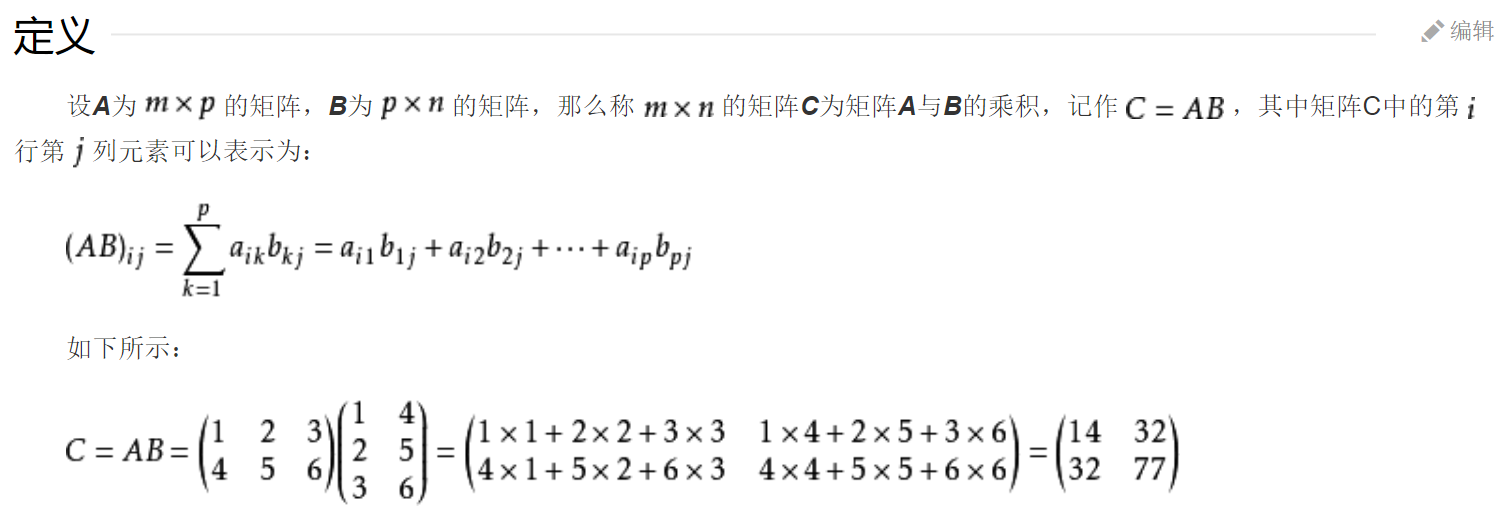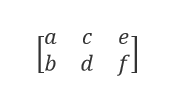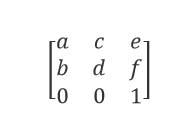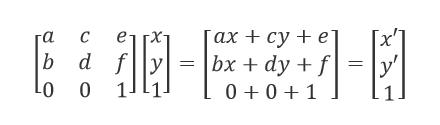## 平移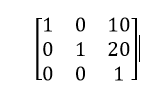transform: matrix(1,0,0,1,10,20); // a=1,c=0,e=10,b=0,d=1,f=20


transform: matrix(1,0,0,1,e,f); // 相当于 transform: translate(e,f);


## 放缩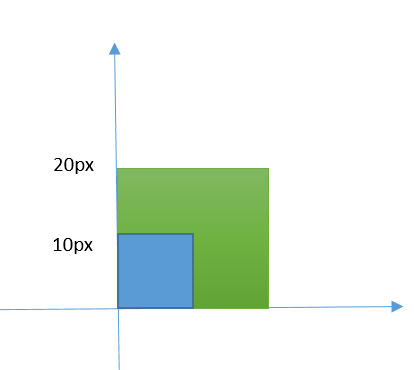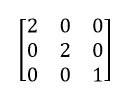transform: matrix(2,0,0,2,0,0); // a=2,c=0,e=0,b=0,d=2,f=0


transform: matrix(a,0,0,d,0,0); // 相当于 transform: scale(a,d)


## 旋转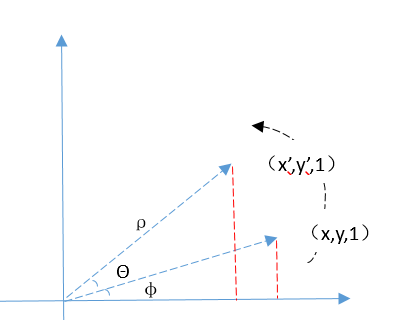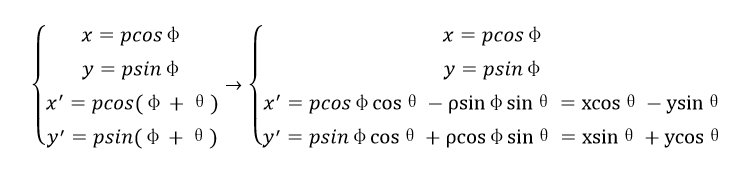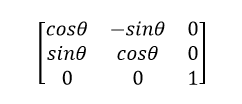q=DCBAp => q=(DCBA)p=Mp


## 斜切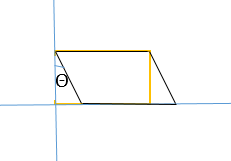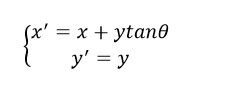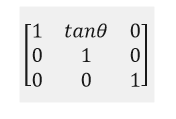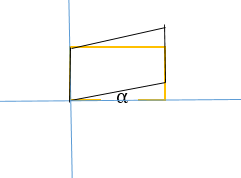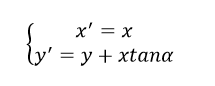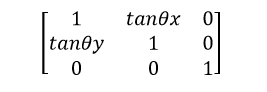【思考】我们之前说过，任何二维组合变换均可分解为多个基本变换的乘积，为什么这里不是将两个扭曲矩阵相乘呢？（先沿x轴扭曲，再沿y轴扭曲？
emmm，是这样的，我们这里的计算都是相对最初始的图形，如果用两个矩阵相乘，则是使初始图形沿x轴扭曲θx度后得到的新图形再对y轴扭曲θy度！！如果要达到我们想要的效果，就只能重新计算沿y轴的扭曲角度！！

• 平移，只需要控制最后两个参数e、f，a、d参数为1
transform: matrix(1,0,0,1,e,f);
// 等同于
transform: translate(e,f);

• 放缩，只需要控制a、d参数，其它为0
transform: matrix(a,0,0,d,0,0);
// 等同于
transform: scale(a,d);

• 旋转，只需要控制a、b、c、d参数
transform: matrix(cosθ, sinθ, -sinθ, cosθ)；
// 等同于
rtransform: rotate(θdeg)

• 斜切
transform: matrix(1, tanθy, tanθx, 1, 0, 0);
// 等同于
transform: skew(θx, θy);

• 复合变换
如以下这种类型，先旋转再缩放再扭曲，如何用矩阵得到呢？
transform: rotate(360deg) scale(2,2) skew(10deg,5deg);


【什么时候我们用矩阵？】

• 需求说：请把这些点沿着y轴取对称。 你：简单
x’ = ax+cy+e = -x，y’ = bx+dy+f = y嘛，一番心算后你得到
transform: matrix(-1,0,0,1,0,0);

• 需求说：算了，还是对y=x取对称吧。你：简单，先整体旋转逆时针旋转45°，再对y轴取对称，再整体顺时针旋转45°（45°）就好了嘛~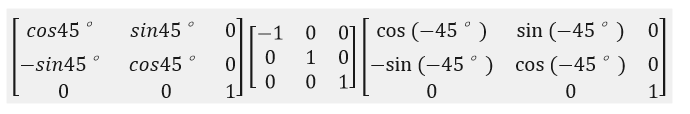(上图右侧表示逆时针旋转)
transform: matrix(0,1,1,0,0,0);

用我们的高中数学来验证一波，点(a,b)关于y=x轴取对称，得到的点应该为（b,a）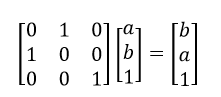验证正确！
• 需求犹豫了0.5s，哎呀还是对y=kx取对称吧…
• 需求摇摇头说，对y=kx+b取对称吧…

1. 两个点关于一条直线对称，那么这两个点的连线的中点必在该直线上
2. 两个点的连线所在直线的斜率与该直线斜率的乘积为-1（因为垂直）
3. 可以列出两个方程，求出对称点！
4. 对称点都求出来了，矩阵也就出来了~
• 现在请看官使用rotate、scale、skew、translate实现上述需求~

【demo】使用css3画一个立方体